# Lunch

Lunch is given to seniors from 12:15 to 12:40 during the Coronavirus pandemic. What angle will the minute hand of clock describe during this time?

A =  150 °

### Step-by-step explanation: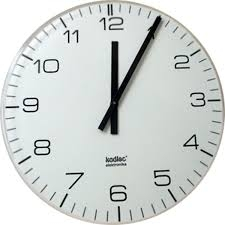Did you find an error or inaccuracy? Feel free to write us. Thank you!Tips to related online calculators
Need help to calculate sum, simplify or multiply fractions? Try our fraction calculator.
Do you want to convert time units like minutes to seconds?

## Related math problems and questions:

• Time clockWhat are the angle of the hour hand and the minute hand if it is 0:40?
• Minute handWhat is the distance the minute hand of the clock travels in 12 minutes, if the diameter of the clock is 30 cm and the hand extends to a distance of 2 cm from the edge of the clock?
• Minute hand v2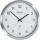In how many minutes describe the minute hand angle 60 degrees?
• Minute-hand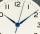How long distance will travel a large (minute) hand on the clock for 48 minutes, if its length is 56 cm?
• The small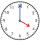The small hand of the wall clock is 15 cm long. What a long journey the end of the hour hand in 15 minutes will describe. (b - in 2 hours)?
• HandsThe clock shows 12 hours. After how many minutes will angle between the hour and minute hand 90°? Consider the continuous movement of both hands hours.
• Lunch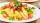Jane eats whole lunch for the 30 minutes. Which part of the lunch is eaten in 180 seconds?
• Little hand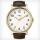What angle shifted little hand on the clock after one hour and 38 minutes?
• Look at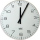Look at the time on the clock now. Information The short hour hand is between 9 and 10. The long minute hand points to 5. Question Second-graders go to recess in 15 minutes. What time will it be then?
• Clock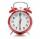What distance will pass end of 8 cm long hour hand for 15 minutes?
• Speed of clockIn how many minutes will the hour hand pass a straight angle and a right angle?
• Clock hands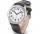The hands-on clock shows the time 12 hours and 2 minutes. Calculate the size of an acute angle between clock hands three hours later.
• Clocks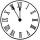What distance will describe the tip of minute hand 6 cm long for 20 minutes when we know the starting position with finally enclose hands each other 120°?
• Special watchFero bought a special watch on the market. They have only one (minute) hand and a display that shows which angle between the hour and minute hand. How many hours was his watch shown - the minute hand points to number 2; the display shows 125°?
• TimeWrite time in minutes rounded to one decimal place: 3 h 48 m 45 s.
• Minute angle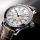Determine size of angle, which takes minute hand for 75 minutes.
• Speed of Slovakian trainsRudolf decided to take the train from the station 'Ostratice' to 'Horné Ozorovce'. In the train timetables found train Os 5409 : km 0 Chynorany 15:17 5 Ostratice 15:23 15:23 8 Rybany 15:27 15:27 10 Dolné Naštice 15:31 15:31 14 Bánovce nad Bebravou 15:35 1